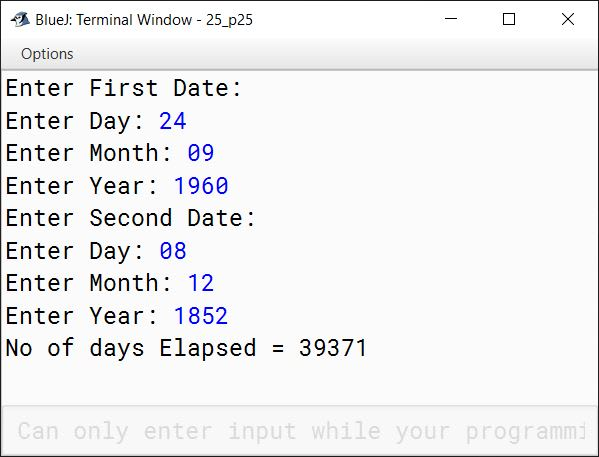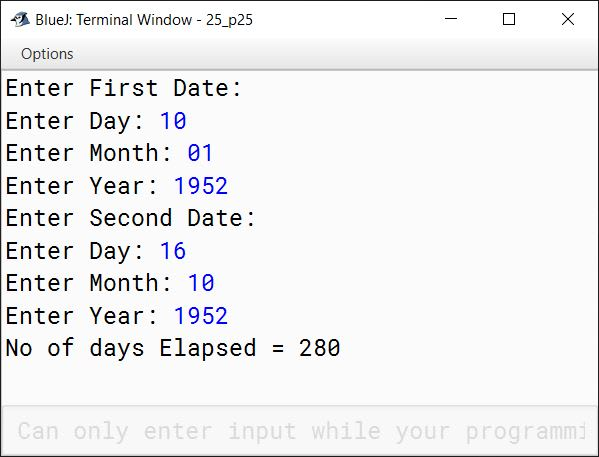# Arrays

## Solutions to Unsolved Programs

#### Question 1

Write a program to input and store n integers (n > 0) in a single subscripted variable and print each number with its frequency. The output should contain number and its frequency in two different columns.

Sample Input:

 12 20 14 12 12 20 16 16 14 14 12 20 18 18

Sample Output:

NumberFrequency
124
143
162
182
203

Solution

```import java.util.Scanner;

public class KboatSDAFrequency
{
public static void main(String args[]) {
Scanner in = new Scanner(System.in);
System.out.print("Enter n: ");
int n = in.nextInt();

if (n <= 0) {
System.out.println("Invalid Input! n should be greater than 0.");
return;
}

int arr[] = new int[n];

System.out.println("Enter array elements:");
for (int i = 0; i < n; i++) {
arr[i] = in.nextInt();
}

//Sort the array
for (int i = 0; i < n - 1; i++) {
for (int j = 0; j < n - i - 1; j++) {
if (arr[j] > arr[j + 1]) {
int t = arr[j];
arr[j] = arr[j+1];
arr[j+1] = t;
}
}
}

System.out.println("Number\tFrequency");
int count = 0;
for (int i = 0; i < n - 1; i++) {
count++;
if (arr[i] != arr[i + 1]) {
System.out.println(arr[i] + "\t" + count);
count = 0;
}
}

//Print frequency of last element
count++;
System.out.println(arr[n - 1] + "\t" + count);
}
}```
##### Output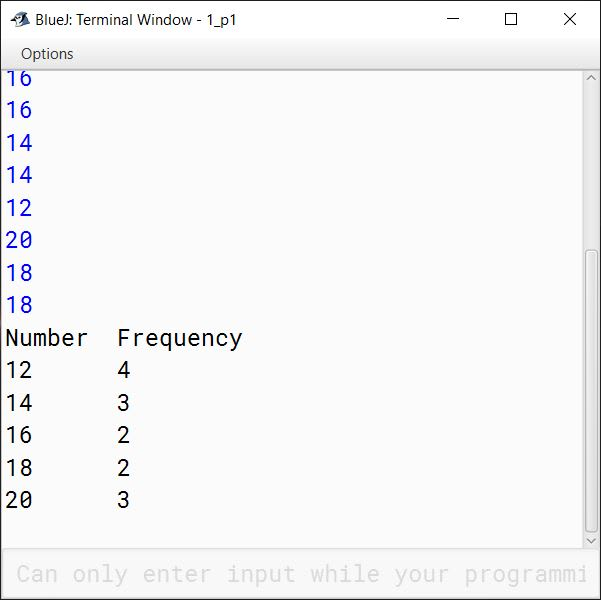#### Question 2

Write a program to accept a set of n integers (where n > 0) in a single dimensional array. Arrange the elements of the array such that the lowest number appears in the centre of the array, next lower number in the right cell of the centre, next lower in the left cell of the centre and so on... . The process will stop when the highest number will set in its appropriate cell. Finally, display the array elements.

Assume that the memory space is less. Hence, you don't need to create extra array for the aforesaid task.

Example:

Input: 1 2 3 4 5
Output: 5 3 1 2 4

Input: 11 12 31 14 5
Output: 31 12 5 11 14

Solution

```import java.util.Scanner;

public class KboatSDAArrange
{
public static void main(String args[]) {
Scanner in = new Scanner(System.in);
System.out.print("Enter n: ");
int n = in.nextInt();

if (n <= 0) {
System.out.println("Invalid Input! n should be greater than 0.");
return;
}

int arr[] = new int[n];
System.out.println("Enter array elements:");
for (int i = 0; i < n; i++) {
arr[i] = in.nextInt();
}

/*
* Steps to arrange the array:
* 1. Sort the array
* {5, 11, 12, 14, 31}
* 2. Get elements at odd indexes in the array
* to the right
* {5, 12, 31, 11, 14}
* 3. Reverse the sub-array from 0 to (n-1) / 2
* {31, 12, 5, 11, 14}
*/

//Step 1: Sort the array
for (int i = 0; i < n - 1; i++) {
for (int j = 0; j < n - i - 1; j++) {
if (arr[j] > arr[j + 1]) {
int t = arr[j];
arr[j] = arr[j+1];
arr[j+1] = t;
}
}
}

//Step 2: Get elements at odd indexes to the right
int endIdx = n - 1;
int startIdx;

if (n % 2 == 0) {
startIdx = n - 1;
}
else {
startIdx = n - 2;
}

while (startIdx > 0) {
int t = arr[startIdx];
int idx = startIdx;

while (idx != endIdx) {
arr[idx] = arr[idx + 1];
idx++;
}

arr[idx] = t;
startIdx -= 2;
endIdx -= 1;
}

//Step 3: Reverse the sub-array from 0 to (n-1) / 2
for (int i = 0, j = (n - 1) / 2; i < j; i++, j--) {
int t = arr[i];
arr[i] = arr[j];
arr[j] = t;
}

//Print the final array
for (int i = 0; i < n; i++) {
System.out.print(arr[i] + " ");
}
}
}```
##### Output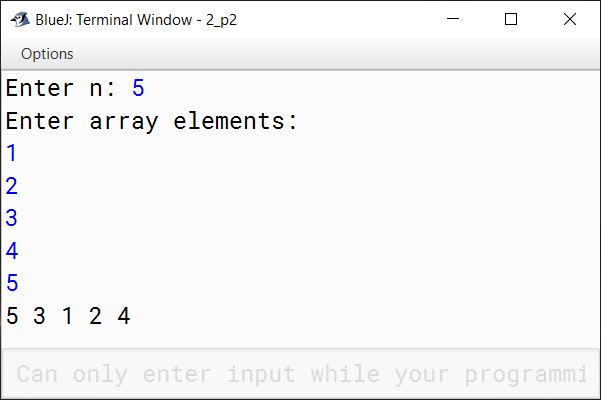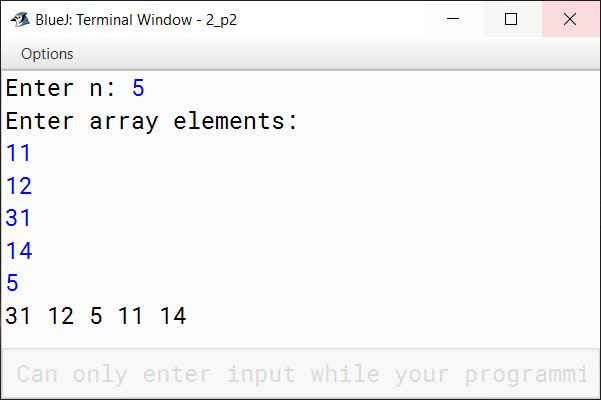#### Question 3

A bank intends to design a program to display the denomination of an input amount, up to 5 digits. The available denomination with the bank are of rupees 2000, 500, 200, 100, 50, 20, 10 and 1.

Design a program to accept the amount from the user and display the break-up in descending order of denominations. (i.e., preference should be given to the highest denomination available) along with the total number of notes.

[Note: Only the denomination used should be displayed].

Also print the amount in words according to the digits.

Example 1:

Input:
14836

Output:
One Four Eight Three Six
Denomination:
2000 * 7 = 14000
500 * 1 = 500
200 * 1 = 200
100 * 1 = 100
20 * 1 = 20
10 * 1 = 10
1 * 6 = 6

Example 2:

Input:
235001

Output:
Invalid Amount

Solution

```import java.util.Scanner;

public class KboatDenominations
{
public static void main(String args[]) {
Scanner in = new Scanner(System.in);
System.out.print("Enter the amount: ");
int amt = in.nextInt();

if (amt > 99999) {
System.out.println("Invalid Amount");
return;
}

String amtInWords = getAmtInWords(amt);
System.out.println(amtInWords);
System.out.println("Denomination:");

int notes[] = {2000, 500, 200, 100, 50, 20, 10, 1};
int t = amt;
for (int i = 0; i < notes.length; i++) {
int c = t / notes[i];
if (c != 0)
System.out.println(notes[i] + "\t*\t"
+ c + "\t=\t" + (c * notes[i]));
t = t % notes[i];
}
}

public static String getAmtInWords(int amt) {
StringBuffer sb = new StringBuffer();

while (amt != 0) {
int d =  amt % 10;
amt /= 10;
switch (d) {
case 0:
sb.insert(0, "Zero ");
break;

case 1:
sb.insert(0, "One ");
break;

case 2:
sb.insert(0, "Two ");
break;

case 3:
sb.insert(0, "Three ");
break;

case 4:
sb.insert(0, "Four ");
break;

case 5:
sb.insert(0, "Five ");
break;

case 6:
sb.insert(0, "Six ");
break;

case 7:
sb.insert(0, "Seven ");
break;

case 8:
sb.insert(0, "Eight ");
break;

case 9:
sb.insert(0, "Nine ");
break;

default:
System.out.println("Invalid digit");
}
}

return sb.toString();
}
}```
##### Output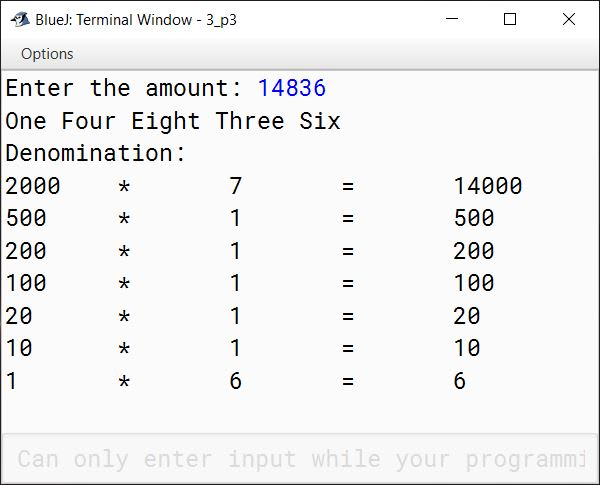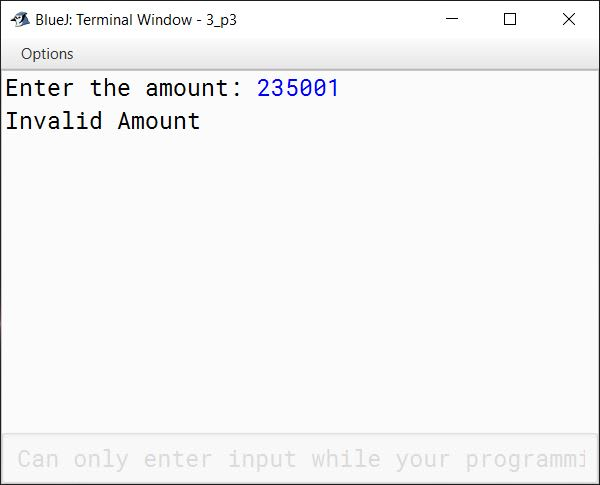#### Question 4

The encryption of letters are to be done as follows:

A = 1
B = 2
C = 3 . . .
Z = 26

The potential of a word is found by adding the encrypted value of the letters.

Example: KITE
Potential = 11 + 9 + 20 + 5 = 45

Accept a sentence which is terminated by either " . " , " ? " or " ! ". Each word of sentence is separated by single space. Decode the words according to their potential and arrange them in alphabetical order increasing order of their potential. Output the result in the format given below:

Example 1

Input:
THE SKY IS THE LIMIT.

Potential:
THE = 33
SKY = 55
IS = 28
THE = 33
LIMIT = 63

Output:
IS THE THE SKY LIMIT

Example 2

Input:
LOOK BEFORE YOU LEAP.

Potential:
LOOK = 53
BEFORE = 51
YOU = 61
LEAP = 34

Output:
LEAP BEFORE LOOK YOU

Solution

```import java.util.*;

public class KboatWordPotential
{
public static void main(String args[]) {
Scanner in = new Scanner(System.in);
System.out.println("ENTER THE SENTENCE:");
String ipStr = in.nextLine();
int len = ipStr.length();

char lastChar = ipStr.charAt(len - 1);
if (lastChar != '.'
&& lastChar != '?'
&& lastChar != '!') {
System.out.println("INVALID INPUT");
return;
}

String str = ipStr.substring(0, len - 1);
StringTokenizer st = new StringTokenizer(str);

int wordCount = st.countTokens();
String strArr[] = new String[wordCount];
int potArr[] = new int[wordCount];

for (int i = 0; i < wordCount; i++) {
strArr[i] = st.nextToken();
potArr[i] = computePotential(strArr[i]);
}

System.out.println("Potential:");
for (int i = 0; i < wordCount; i++) {
System.out.println(strArr[i] + " = " + potArr[i]);
}

/*
* Sort potential array and words array
* as per potential array
*/
for (int i = 0; i < wordCount - 1; i++) {
for (int j = 0; j < wordCount - i - 1; j++) {
if (potArr[j] > potArr[j+1]) {
int t = potArr[j];
potArr[j] = potArr[j+1];
potArr[j+1] = t;

String temp = strArr[j];
strArr[j] = strArr[j+1];
strArr[j+1] = temp;
}
}
}

System.out.println("Sorted Sentence");
for (int i = 0; i < wordCount; i++) {
System.out.print(strArr[i] + " ");
}
}

public static int computePotential(String word) {
String str = word.toUpperCase();
int p = 0;
int l = str.length();
/*
* Substracting 64 from ASCII Value of
* letter gives the proper potentials:
* A => 65 - 64 = 1
* B => 66 - 64 = 2
* ..
* ..
*/
for (int i = 0; i < l; i++) {
p += str.charAt(i) - 64;
}

return p;
}
}```
##### Output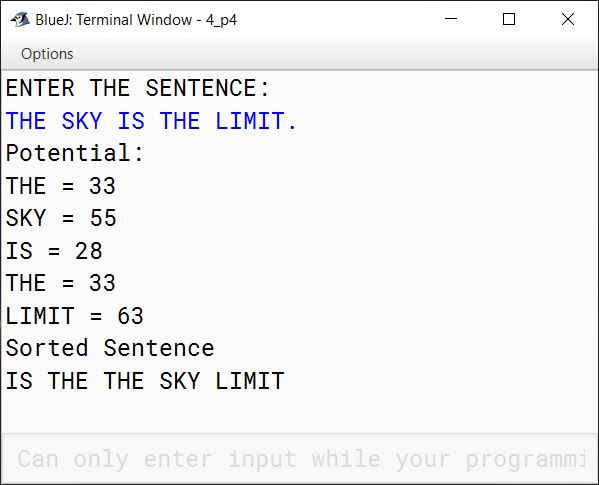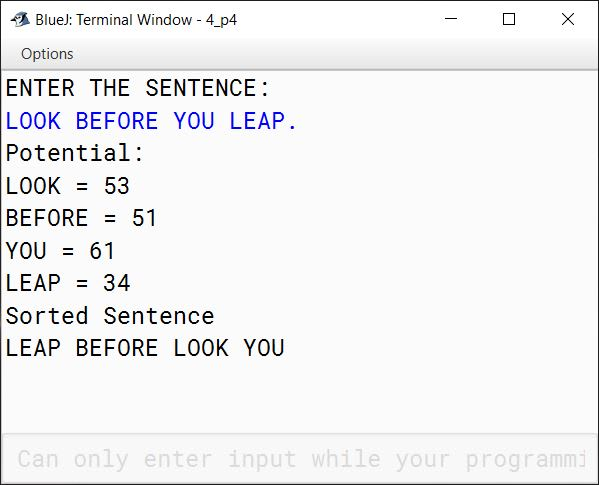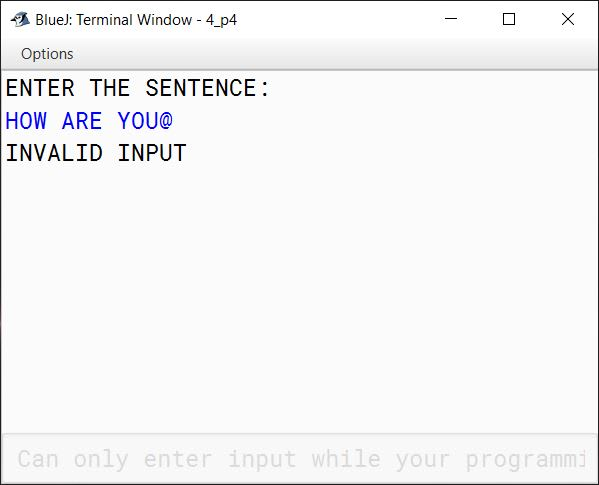#### Question 5

A company manufactures packing cartons in four sizes, i.e. cartons to accommodate 6 boxes, 12 boxes, 24 boxes and 48 boxes. Design a program to accept the number of boxes to be packed (N) by the user (maximum up to 1000 boxes) and display the break-up of the cartons used in descending order of capacity (i.e. preference should be given to the highest capacity available, and if boxes left are less than 6, an extra carton of capacity 6 should be used.)

Test your program with the following data and some random data:

Example 1

INPUT:
N = 726

OUTPUT:
48 * 15 = 720
6 * 1 = 6
Remaining boxes = 0
Total number of boxes = 726
Total number of cartons = 16

Example 2

INPUT:
N = 140

OUTPUT:
48 * 2 = 96
24 * 1 = 24
12 * 1 = 12
6 * 1 = 6
Remaining boxes = 2 * 1 = 2
Total number of boxes = 140
Total number of cartons = 6

Example 3

INPUT:
N = 4296

OUTPUT:
INVALID INPUT

Solution

```import java.util.Scanner;

public class CartonBoxes
{
public static void main(String args[]) {
Scanner in = new Scanner(System.in);

System.out.print("Enter number of boxes (N): ");
int n = in.nextInt();

if (n < 1 || n > 1000) {
System.out.println("INVALID INPUT");
return;
}

int cartonSizes[] = {48, 24, 12, 6};

int total = 0;
int t = n;
for (int i = 0; i < cartonSizes.length; i++) {
int cartonCount = t / cartonSizes[i];
t = t % cartonSizes[i];
total += cartonCount;
if (cartonCount != 0) {
System.out.println(cartonSizes[i] + " * " + cartonCount +
" = " + (cartonSizes[i] * cartonCount));
}
}

/*
* This if check is for the case when
* boxes left are less than 6. We need
* one more carton of capacity 6 in this
* case so total is incremented by 1.
*/
if (t != 0) {
System.out.println("Remaining boxes = " + t
+ " * 1 = " + t);
total++;
}
else {
System.out.println("Remaining boxes = 0");
}

System.out.println("Total number of boxes = " + n);
System.out.println("Total number of cartons = " + total);
}
}```
##### Output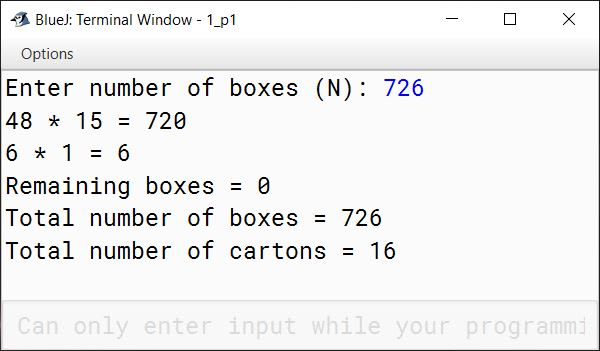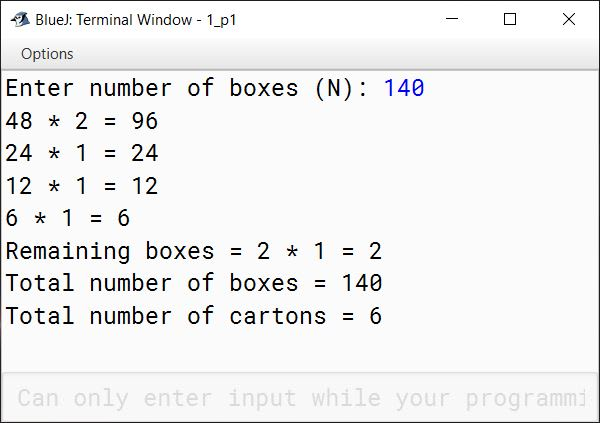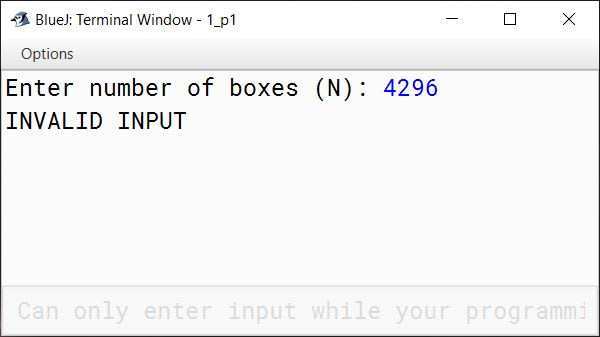#### Question 6

Given a square matrix M[][] of order 'n'. The maximum value possible for 'n' is 10. Accept three different characters from the keyboard and fill the array according to the output shown in the examples. If the value of n exceeds 10 then an appropriate message should be displayed.

Example 1

Enter Size: 4

Input:
First Character '*'
Second Character '?'
Third Character '#'

Output:
# * * #
? # # ?
? # # ?
# * * #

Example 2

Enter Size: 5

Input:
First Character '\$'
Second Character '!'
Third Character '@'

Output:
@ \$ \$ \$ @
! @ \$ @ !
! ! @ ! !
! @ \$ @ !
@ \$ \$ \$ @

Example 3

Enter Size: 65

Output:
Size out of Range

Solution

```import java.util.Scanner;

public class KboatCharMatrix
{
public static void main(String args[]) {
Scanner in = new Scanner(System.in);
System.out.print("Enter size: ");
int n = in.nextInt();

if (n < 1 || n > 10) {
System.out.println("Size out of Range");
return;
}

System.out.print("First Character: ");
char ch1 = in.next().charAt(0);
System.out.print("Second Character: ");
char ch2 = in.next().charAt(0);
System.out.print("Third Character: ");
char ch3 = in.next().charAt(0);

char m[][] = new char[n][n];
char x = ch2;
for (int i = 0; i < n; i++) {
for (int j = 0; j < n; j++) {
if (i == j || (i + j) == n - 1) {
m[i][j] = ch3;
}
else if (i == 0 || i == n - 1) {
m[i][j] = ch1;
}
else if (j == 0 || j == n - 1 || i % 2 == 0) {
m[i][j] = ch2;
}
else {
m[i][j] = ch1;
}
}
}

System.out.println("OUTPUT:");
for (int i = 0; i < n; i++) {
for (int j = 0; j < n; j++) {
System.out.print(m[i][j] + "\t");
}
System.out.println();
}
}
}```
##### Output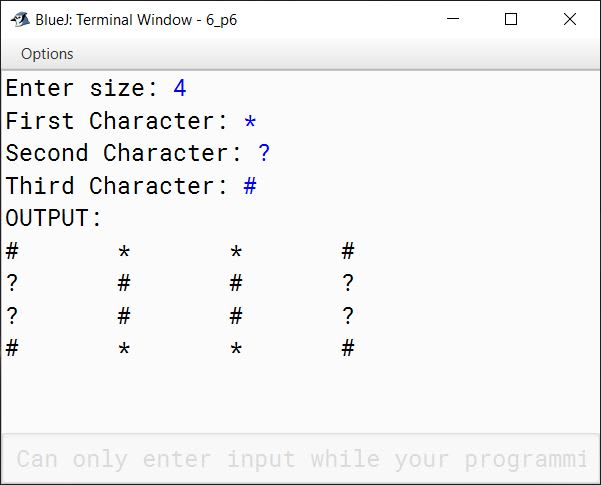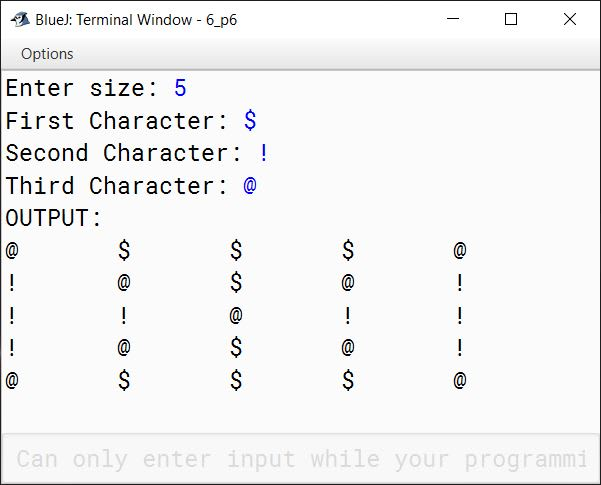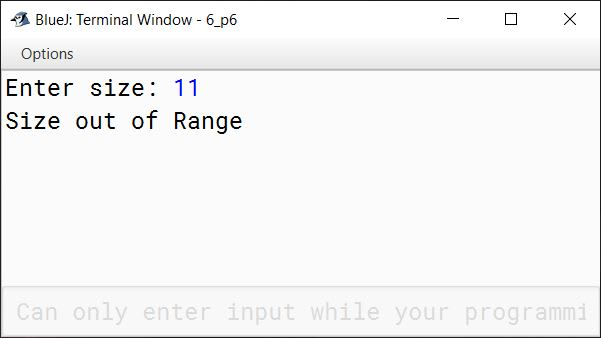#### Question 7

The result of a quiz competition is to be prepared as follows:

The quiz has five questions with four multiple choices (A, B, C, D), with each question carrying 1 mark for the correct answer. Design a program to accept the number of participants N such that N must be greater than 3 and less than 11. Create a double-dimensional array of size (Nx5) to store the answers of each participant row-wise. Calculate the marks for each participant by matching the correct answer stored in a single-dimensional array of size 5. Display the scores for each participant and also the participant(s) having the highest score.

Example: If the value of N = 4, then the array would be:

Q1Q2Q3Q4Q5
Participant 1ABBCA
Participant 3AABAC
Participant 4DCCAB
 Key to the question: D C C B A

Note: Array entries are line fed (i.e. one entry per line)

Test your program for the following data and some random data.

Example 1

INPUT:
N = 5
Participant 1 D A B C C
Participant 2 A A D C B
Participant 3 B A C D B
Participant 4 D A D C B
Participant 5 B C A D D
Key: B C D A A

OUTPUT:
Scores:
Participant 1 = 0
Participant 2 = 1
Participant 3 = 1
Participant 4 = 1
Participant 5 = 2
Highest Score:
Participant 5

Example 2

INPUT:
N = 4
Participant 1 A C C B D
Participant 2 B C A A C
Participant 3 B C B A A
Participant 4 C C D D B
Key: A C D B B

OUTPUT:
Scores:
Participant 1 = 3
Participant 2 = 1
Participant 3 = 1
Participant 4 = 3
Highest Score:
Participant 1
Participant 4

Example 3

INPUT:
N = 12

OUTPUT:
INPUT SIZE OUT OF RANGE.

Solution

```import java.util.Scanner;

public class QuizCompetition
{
public static void main(String args[]) {
Scanner in = new Scanner(System.in);
System.out.print("Enter the Number of Participants (N): ");
int n = in.nextInt();

if (n <= 3 || n >= 11) {
System.out.println("INPUT SIZE OUT OF RANGE.");
return;
}

char key[] = new char;

for (int i = 0; i < n; i++) {
System.out.println("Participant " + (i+1));
for (int j = 0; j < 5; j++) {
}
}

for (int i = 0; i < 5; i++) {
key[i] = in.next().charAt(0);
}

int hScore = 0;
int score[] = new int[n];

System.out.println("Scores:");
for (int i = 0; i < n; i++) {
for (int j = 0; j < 5; j++) {
score[i]++;
}
}

if (score[i] > hScore) {
hScore = score[i];
}

System.out.println("Participant " + (i+1) + " = " + score[i]);
}

System.out.println("Highest Score:");
for (int i = 0; i < n; i++) {
if (score[i] == hScore) {
System.out.println("Participant " + (i+1));
}
}
}
}```
##### Output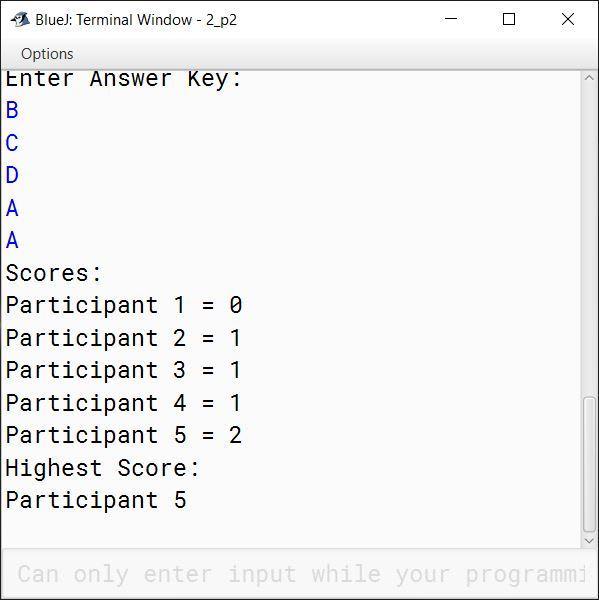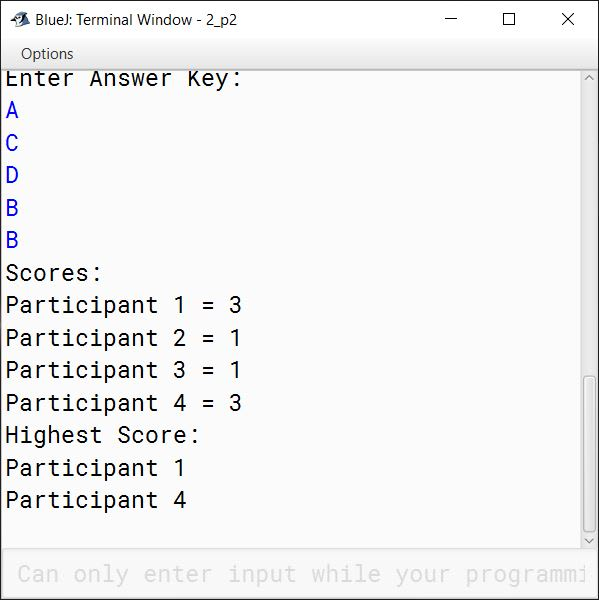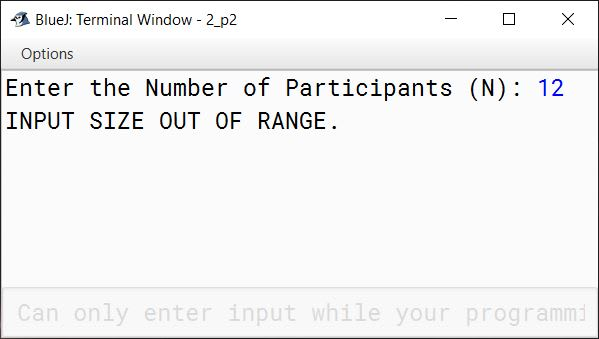#### Question 8

Write a Program in Java to input elements in a 2D square matrix and check whether it is a Lower Triangular Matrix or not.

Lower Triangular Matrix: A Lower Triangular matrix is a square matrix in which all the entries above the main diagonal [] are zero. The entries below or on the main diagonal must be non zero values.

Enter the size of the matrix: 4
The Matrix is:
5 0 0 0
3 1 0 0
4 9 4 0
6 8 7 2

The Matrix is Lower Triangular

Solution

```import java.util.Scanner;

public class KboatLowerTriangularMatrix
{
public static void main(String args[]) {
Scanner in = new Scanner(System.in);
System.out.print("Enter the size of the matrix: ");
int n = in.nextInt();

int arr[][] = new int[n][n];

System.out.println("Enter elements of the matrix: ");
for (int i = 0; i < n; i++) {
System.out.println("Enter Row "+ (i+1) + " :");
for (int j = 0; j < n; j++) {
arr[i][j] = in.nextInt();
}
}

System.out.println("The Matrix is:");
for (int i = 0; i < n; i++) {
for (int j = 0; j < n; j++) {
System.out.print(arr[i][j] + "\t");
}
System.out.println();
}

boolean isTriangular = true;
for (int i = 0; i < n; i++) {
for (int j = 0; j < n; j++) {
if ((i < j && arr[i][j] != 0)
|| (i >= j && arr[i][j] == 0)) {
isTriangular = false;
break;
}
}

if (!isTriangular) {
break;
}
}

if (isTriangular) {
System.out.println("The Matrix is Lower Triangular");
}
else {
System.out.println("The Matrix is not Lower Triangular");
}
}
}```
##### Output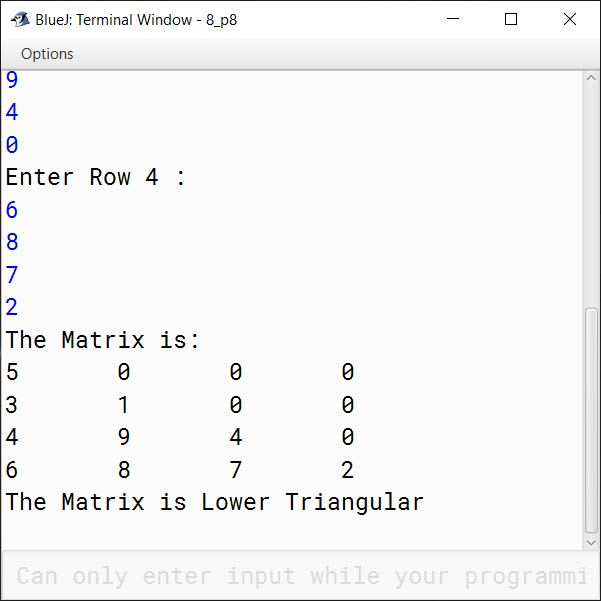#### Question 9

Write a Program in Java to input elements in a 2-D square matrix and check whether it is a Scalar Matrix or not.

Scalar Matrix: A scalar matrix is a diagonal matrix where the left diagonal elements are same.

The Matrix is:
5 0 0 0
0 5 0 0
0 0 5 0
0 0 0 5

The Matrix is Scalar

Solution

```import java.util.Scanner;

public class KboatScalarMatrix
{
public static void main(String args[]) {
Scanner in = new Scanner(System.in);
System.out.print("Enter the size of the matrix: ");
int n = in.nextInt();

int arr[][] = new int[n][n];

System.out.println("Enter elements of the matrix: ");
for (int i = 0; i < n; i++) {
System.out.println("Enter Row "+ (i+1) + " :");
for (int j = 0; j < n; j++) {
arr[i][j] = in.nextInt();
}
}

System.out.println("The Matrix is:");
for (int i = 0; i < n; i++) {
for (int j = 0; j < n; j++) {
System.out.print(arr[i][j] + "\t");
}
System.out.println();
}

boolean isScalar = true;
int x = arr;
for (int i = 0; i < n; i++) {
for (int j = 0; j < n; j++) {
if ((i == j && arr[i][j] != x)
|| ( i != j && arr[i][j] != 0)) {
isScalar = false;
break;
}
}

if (!isScalar) {
break;
}
}

if (isScalar) {
System.out.println("The Matrix is Scalar");
}
else {
System.out.println("The Matrix is not Scalar");
}
}
}```
##### Output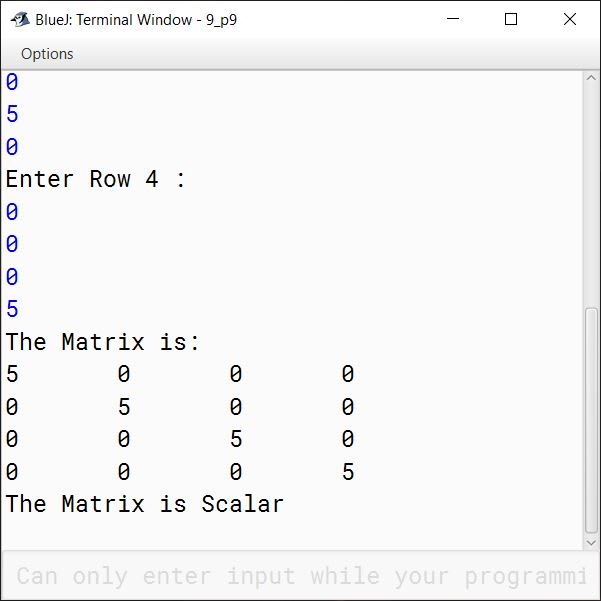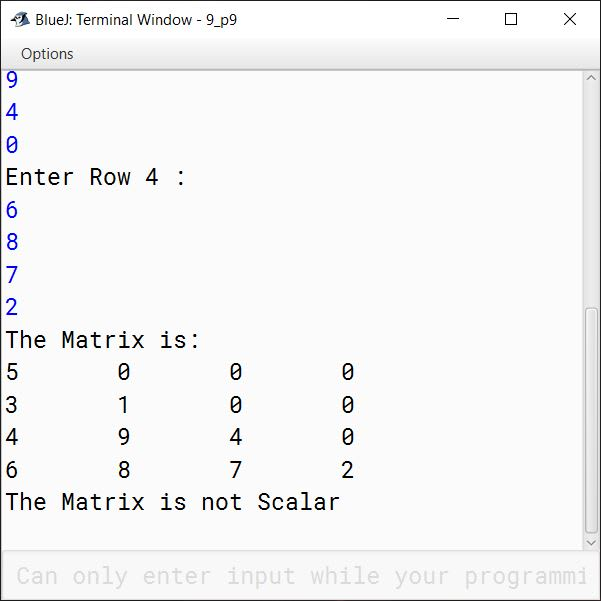#### Question 10

A square matrix is the matrix in which number of rows equals the number of columns. Thus, a matrix of order n*n is called a Square Matrix.

Write a program in Java to create a double dimensional array of size nxn matrix form and fill the numbers in a circular fashion (anticlock-wise) with natural numbers from 1 to n2, taking n as an input. The filling of the elements should start from outer to the central cell.

For example, if n=4, then n2=16, then the array is filled as:

 1 ↓ ↓ 12 ←11 ←10 2 ↓ ↓ 13 16 ↑ 9   ↑ 3 ↓ 14   → 15 ↑ 8   ↑ 4→ 5→ 6→ 7   ↑

Solution

```import java.util.Scanner;

public class KboatMatrixCircularFill
{
public static void main(String args[]) {
Scanner in = new Scanner(System.in);
System.out.print("Enter the size of the matrix: ");
int n = in.nextInt();
int x = 1;
int arr[][] = new int[n][n];

int a = 0;
int b = n - 1;

while (a < n) {

for (int i = a; i <= b; i++) {
arr[i][a] = x++;
}

a++;

for (int i = a; i <= b; i++) {
arr[b][i] = x++;
}

for (int i = b - 1; i >= a - 1; i--) {
arr[i][b] = x++;
}

b--;

for (int i = b; i >= a; i--) {
arr[a-1][i] = x++;
}
}

System.out.println("Circular Matrix Anti-Clockwise:");

for (int i = 0; i < n; i++) {
for (int j = 0; j < n; j++) {
System.out.print(arr[i][j] + "\t");
}
System.out.println();
}
}
}```
##### Output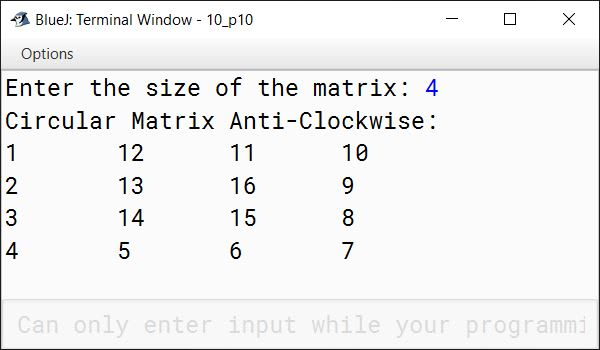#### Question 11

A square matrix is the matrix in which number of rows equals the number of columns. Thus, a matrix of order n*n is called a Square Matrix.

Write a program in Java to create a double dimensional array of size nxn matrix form and fill the cells of matrix in a circular fashion (clock wise) with natural numbers from 1 to n2, taking n as an input. Input n should be an odd number and filling of the elements should start from the central cell.

For example, if n = 5, then n2 = 25, then the array is filled as:

 21 22 23 24 25 20 7 8 9 10 19 6 1 2 11 18 5 4 3 12 17 16 15 14 13

Solution

```import java.util.Scanner;

public class KboatMatrixClockwise
{
public static void main(String args[]) {
Scanner in = new Scanner(System.in);
System.out.print("Enter the size of the matrix: ");
int n = in.nextInt();

if (n % 2 == 0) {
System.out.println("Invalid Input! Size must be an odd number");
return;
}

int val  = 1;
int arr[][] = new int[n][n];

int x = n / 2;
int y = n / 2;
int d = 0;
int c = 0;
int s = 1;

for (int k = 1; k <= (n - 1); k++) {
for (int j = 0; j < (k < n - 1 ? 2 : 3); j++) {
for (int i = 0; i < s; i++) {
arr[x][y] = val++;

switch (d) {
case 0:
y = y + 1;
break;

case 1:
x = x + 1;
break;

case 2:
y = y - 1;
break;

case 3:
x = x - 1;
break;
}
}

d = (d + 1) % 4;
}

s = s + 1;
}

arr[n-1] = val;

System.out.println("Circular Matrix Clockwise:");

for (int i = 0; i < n; i++) {
for (int j = 0; j < n; j++) {
System.out.print(arr[i][j] + "\t");
}
System.out.println();
}
}
}```
##### Output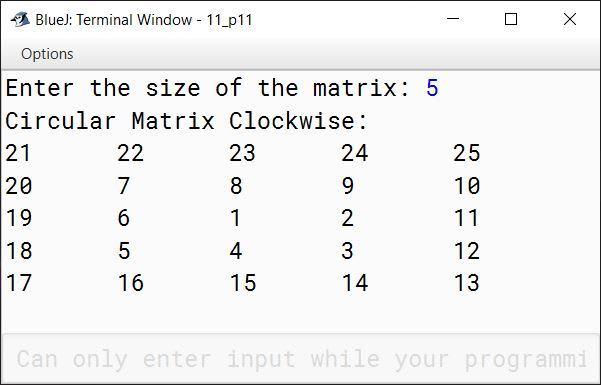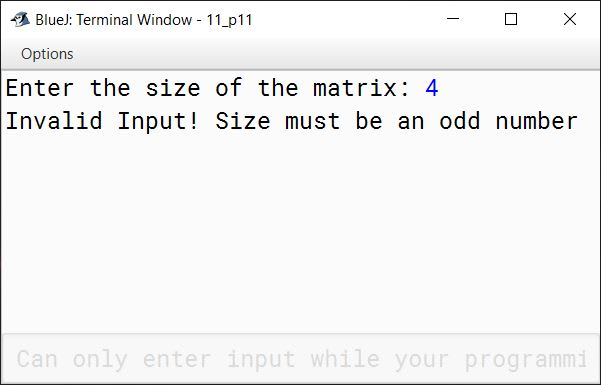#### Question 12

Write a program in Java create a double dimensional array of size nxn matrix form and fill the numbers in a circular fashion (anticlock-wise) with natural numbers from 1 to n2, as illustrated below:

For example, if n = 5, then n2 = 25, then the array is filled as:

 21 20 19 18 17 22 7 6 5 16 23 8 1 4 15 24 9 2 3 14 25 10 11 12 13

Solution

```import java.util.Scanner;

public class KboatMatrixAntiClockwise
{
public static void main(String args[]) {
Scanner in = new Scanner(System.in);
System.out.print("Enter the size of the matrix: ");
int n = in.nextInt();

if (n % 2 == 0) {
System.out.println("Invalid Input! Size must be an odd number");
return;
}

int val  = 1;
int arr[][] = new int[n][n];

int x = n / 2;
int y = n / 2;
int d = 1;
int c = 0;
int s = 1;

for (int k = 1; k <= (n - 1); k++) {
for (int j = 0; j < (k < n - 1 ? 2 : 3); j++) {
for (int i = 0; i < s; i++) {
arr[x][y] = val++;

switch (d) {
case 0:
y = y - 1;
break;

case 1:
x = x + 1;
break;

case 2:
y = y + 1;
break;

case 3:
x = x - 1;
break;
}
}

d = (d + 1) % 4;
}

s = s + 1;
}

arr[n-1] = val;

System.out.println("Circular Matrix AntiClockwise:");

for (int i = 0; i < n; i++) {
for (int j = 0; j < n; j++) {
System.out.print(arr[i][j] + "\t");
}
System.out.println();
}
}
}```
##### Output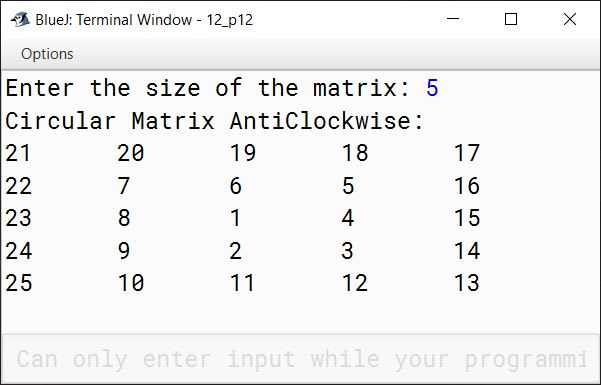#### Question 13

Write a program in Java to enter natural numbers in a double dimensional array mxn (where m is the number of rows and n is the number of columns). Shift the elements of 4th column into the 1st column, the elements of 1st column into the 2nd column and so on. Display the new matrix.

 11 16 7 4 8 10 9 18 9 8 12 15 14 15 13 6 Sample Input
 4 11 16 7 18 8 10 9 15 9 8 12 6 14 15 13 Sample Output

Solution

```import java.util.Scanner;

public class KboatSDAColShift
{
public static void main(String args[]) {
Scanner in = new Scanner(System.in);
System.out.print("Enter number of rows (m): ");
int m = in.nextInt();
System.out.print("Enter number of columns (n): ");
int n = in.nextInt();

int arr[][] = new int[m][n];
int newArr[][] = new int[m][n];

System.out.println("Enter array elements");
for (int i = 0; i < m; i++) {
System.out.println("Enter Row "+ (i+1) + " :");
for (int j = 0; j < n; j++) {
arr[i][j] = in.nextInt();
}
}

System.out.println("Input Array:");
for (int i = 0; i < m; i++) {
for (int j = 0; j < n; j++) {
System.out.print(arr[i][j] + "\t");
}
System.out.println();
}

for (int j = 0; j < n; j++) {
int col = j + 1;
if (col == n) {
col = 0;
}
for (int i = 0; i < m; i++) {
newArr[i][col] = arr[i][j];
}
}

System.out.println("New Shifted Array:");
for (int i = 0; i < m; i++) {
for (int j = 0; j < n; j++) {
System.out.print(newArr[i][j] + "\t");
}
System.out.println();
}
}
}```
##### Output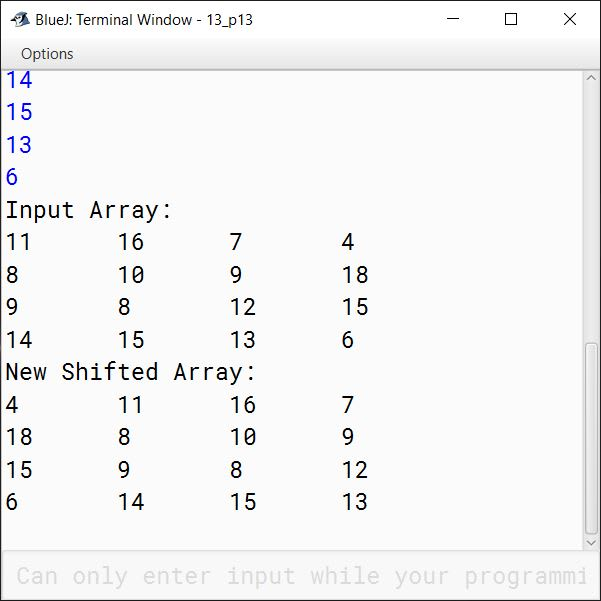#### Question 14

Write a program in Java to enter natural numbers in a double dimensional array m x n (where m is the number of rows and n is the number of columns). Display the new matrix in such a way that the new matrix is the mirror image of the original matrix.

 8 15 9 18 9 10 7 6 10 8 11 13 12 16 17 19 Sample Input
 18 9 15 8 6 7 10 9 13 11 8 10 19 17 16 12 Sample Output

Solution

```import java.util.Scanner;

public class KboatSDAMirrorImage
{
public static void main(String args[]) {
Scanner in = new Scanner(System.in);
System.out.print("Enter number of rows (m): ");
int m = in.nextInt();
System.out.print("Enter number of columns (n): ");
int n = in.nextInt();

int arr[][] = new int[m][n];
int newArr[][] = new int[m][n];

System.out.println("Enter array elements");
for (int i = 0; i < m; i++) {
System.out.println("Enter Row "+ (i+1) + " :");
for (int j = 0; j < n; j++) {
arr[i][j] = in.nextInt();
}
}

System.out.println("Input Array:");
for (int i = 0; i < m; i++) {
for (int j = 0; j < n; j++) {
System.out.print(arr[i][j] + "\t");
}
System.out.println();
}

for (int j = 0; j < n; j++) {
for (int i = 0; i < m; i++) {
newArr[i][n - 1 - j] = arr[i][j];
}
}

System.out.println("Mirror Image Array:");
for (int i = 0; i < m; i++) {
for (int j = 0; j < n; j++) {
System.out.print(newArr[i][j] + "\t");
}
System.out.println();
}
}
}```
##### Output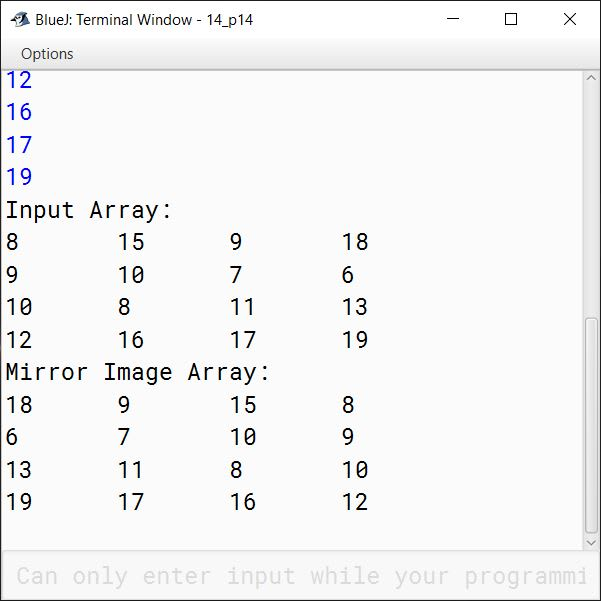#### Question 15

Write a Program in Java to fill a 2D array with the first 'mxn' prime numbers, where 'm' is the number of rows and 'n' is the number of columns.

For example:
If rows = 4 and columns = 5, then the result should be:

 2 3 5 7 11 13 17 19 23 29 31 37 41 43 47 53 59 61 67 71

Solution

```import java.util.Scanner;

public class KboatSDAPrime
{
public static void main(String args[]) {
Scanner in = new Scanner(System.in);
System.out.print("Enter number of rows (m): ");
int m = in.nextInt();
System.out.print("Enter number of columns (n): ");
int n = in.nextInt();

int arr[][] = new int[m][n];
int r = 0, c = 0;
int total = m * n;
int count = 0;
for (int i = 2; count < total; i++) {

int div = 0;
for (int j = 1; j <= i; j++) {
if (i % j == 0) {
div++;
}
}

if (div == 2) {
arr[r][c++] = i;
count++;
if (c == n) {
r++;
c = 0;
}
}

}

System.out.println("Prime Numbers Array:");
for (int i = 0; i < m; i++) {
for (int j = 0; j < n; j++) {
System.out.print(arr[i][j] + "\t");
}
System.out.println();
}
}
}```
##### Output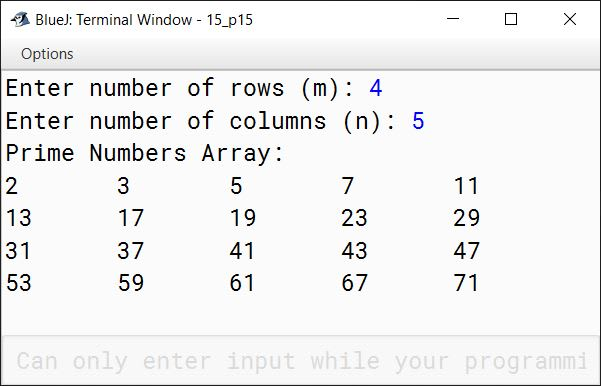#### Question 16

Write a program to create a double dimensional array of size n x m. Input the numbers in first (n-1) x (m-1) cells. Find and place the sum of each row and each column in corresponding cells of last column and last row respectively. Finally, display the array elements along with the sum of rows and columns.

Sample Input

 10 15 16 18 15 14 12 11 11 12 16 17 12 10 14 16

Sample Output

 10 15 16 18 59 15 14 12 11 52 11 12 16 17 56 12 10 14 16 52 48 51 58 62

Solution

```import java.util.Scanner;

public class KboatDDASum
{
public static void main(String args[]) {
Scanner in = new Scanner(System.in);
System.out.print("Enter number of rows (n): ");
int n = in.nextInt();
System.out.print("Enter number of columns (m): ");
int m = in.nextInt();

int arr[][] = new int[n][m];

System.out.println("Enter array elements");
for (int i = 0; i < n - 1; i++) {
System.out.println("Enter Row "+ (i+1) + " :");
for (int j = 0; j < m - 1; j++) {
arr[i][j] = in.nextInt();
}
}

System.out.println("Input Array:");
for (int i = 0; i < n; i++) {
for (int j = 0; j < m; j++) {
System.out.print(arr[i][j] + "\t");
}
System.out.println();
}

//Row-wise & Column-wise Sum
for (int i = 0; i < n - 1; i++) {
int rSum = 0, cSum = 0;
for (int j = 0; j < m - 1; j++) {
rSum += arr[i][j];
cSum += arr[j][i];
}
arr[i][m - 1] = rSum;
arr[n - 1][i] = cSum;
}

System.out.println("Array with Sum:");
for (int i = 0; i < n; i++) {
for (int j = 0; j < m; j++) {
System.out.print(arr[i][j] + "\t");
}
System.out.println();
}
}
}```
##### Output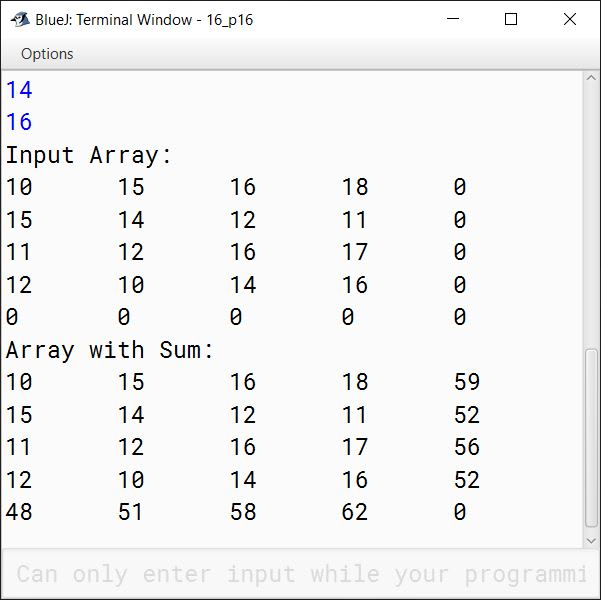#### Question 17

Write a program in Java to create a 4 x 4 matrix. Now, swap the elements of 0th row with 3rd row correspondingly. Display the result after swapping.

Sample Input

 55 33 26 14 81 86 31 10 58 64 17 12 22 14 23 25

Sample Output

 22 14 23 25 81 86 31 10 58 64 17 12 55 33 26 14

Solution

```import java.util.Scanner;

public class KboatDDARowSwap
{
public static void main(String args[]) {
Scanner in = new Scanner(System.in);
int arr[][] = new int;

System.out.println("Enter elements of 4x4 array ");
for (int i = 0; i < 4; i++) {
System.out.println("Enter Row "+ (i+1) + " :");
for (int j = 0; j < 4; j++) {
arr[i][j] = in.nextInt();
}
}

System.out.println("Input Array:");
for (int i = 0; i < 4; i++) {
for (int j = 0; j < 4; j++) {
System.out.print(arr[i][j] + "\t");
}
System.out.println();
}

//Swap 0th and 3rd rows
for (int j = 0; j < 4; j++) {
int t = arr[j];
arr[j] = arr[j];
arr[j] = t;
}

System.out.println("Swapped Array:");
for (int i = 0; i < 4; i++) {
for (int j = 0; j < 4; j++) {
System.out.print(arr[i][j] + "\t");
}
System.out.println();
}
}
}```
##### Output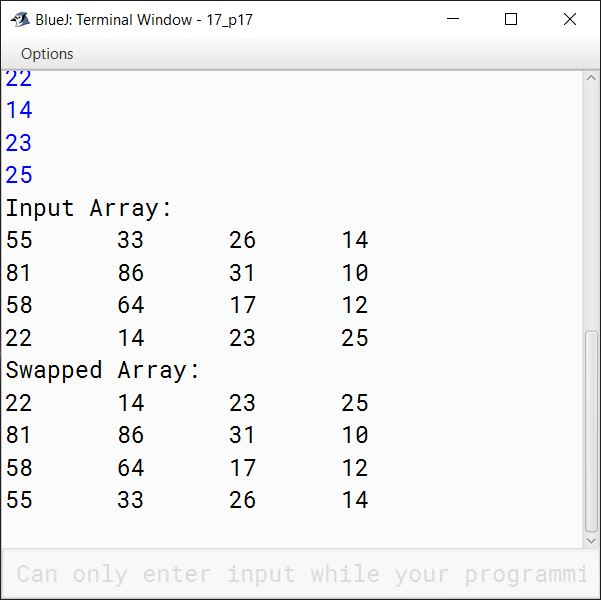#### Question 18

Write a program in Java to store the elements in two different double dimensional arrays (in matrix form) A and B each of order 4 x 4. Find the product of both the matrices and store the result in matrix C. Display the elements of matrix C.

Note:
Two matrixes can be multiplied only if the number of columns of the first matrix must be equal to the number of rows of the second matrix.

Sample Input: Matrix A

 3 2 1 2 6 4 5 0 7 -1 0 2 4 3 1 1

Sample Input: Matrix B

 -2 -4 -1 0 3 6 -5 2 5 3 4 6 0 -2 2 5

Sample Output: Matrix C

 5 -1 -5 20 25 15 -6 38 -17 -38 2 -8 6 3 -13 17

Solution

```import java.util.Scanner;

public class KboatDDAMultiply
{
public static void main(String args[]) {

Scanner in = new Scanner(System.in);

int a[][] = new int;
int b[][] = new int;
int c[][] = new int;

System.out.println("Enter elements of Matrix A");
for (int i = 0; i < 4; i++) {
System.out.println("Enter Row "+ (i+1) + " :");
for (int j = 0; j < 4; j++) {
a[i][j] = in.nextInt();
}
}

System.out.println("Enter elements of Matrix B");
for (int i = 0; i < 4; i++) {
System.out.println("Enter Row "+ (i+1) + " :");
for (int j = 0; j < 4; j++) {
b[i][j] = in.nextInt();
}
}

System.out.println("Input Matrix A:");
printMatrix(a, 4, 4);

System.out.println("Input Matrix B:");
printMatrix(b, 4, 4);

//Multiply the Matrices
for (int i = 0; i < 4; i++) {
for (int j = 0; j < 4; j++) {
for (int k = 0; k < 4; k++) {
c[i][j] += a[i][k] * b[k][j];
}

}
}

System.out.println("Output Matrix C:");
printMatrix(c, 4, 4);
}

public static void printMatrix(int arr[][], int m, int n) {
for (int i = 0; i < m; i++) {
for (int j = 0; j < n; j++) {
System.out.print(arr[i][j] + "\t");
}
System.out.println();
}
}
}```
##### Output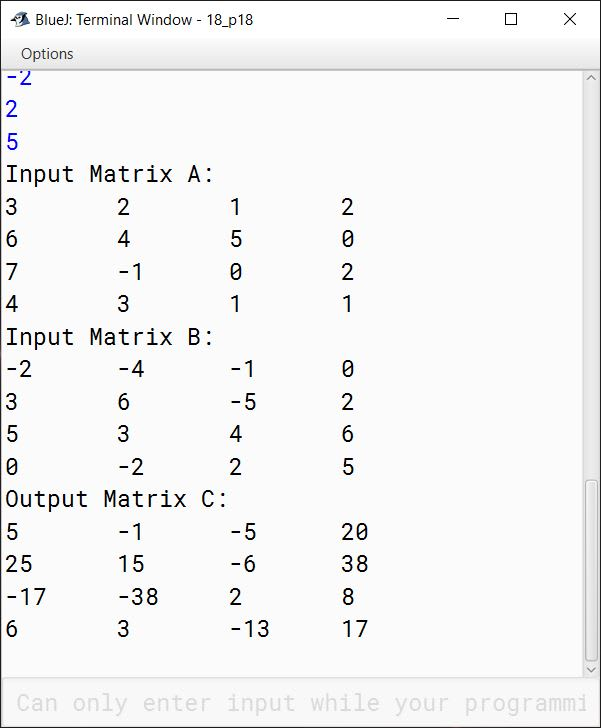#### Question 19

1. Write a program to declare a square matrix A [ ] ] of order N (N<20).
2. Allow the user to input positive integers into this matrix. Perform the following tasks on the matrix.
3. Output the original matrix.
4. Find the SADDLE POINT for the matrix. A saddle point is an element of the matrix such that it is the minimum element for the row and the maximum element for the column to which it belongs. Saddle point for a given matrix is always unique. If the matrix has no saddle point, output the message "NO SADDLE POINT".
5. If the matrix has a saddle point element then sort the elements of the left diagonal in ascending order using insertion sort technique. All other elements should remain unchanged.

Test your program for the following data and some random data:

Sample data:

Input:
n = 4

 Matrix A[ ][ ] 2 5 6 9 8 4 12 3 6 7 3 1 12 24 2 11

Output:

 Matrix A[ ][ ] 2 5 6 9 8 4 12 3 6 7 3 1 12 24 2 11

Matrix after sorting the Principal diagonal:

 2 5 6 9 8 3 12 3 6 7 4 1 12 24 2 11

Input:
n = 3

 Matrix A[ ][ ] 4 6 12 2 8 14 1 3 6

Output:

 Matrix A[ ][ ] 4 6 12 2 8 14 1 3 6

Matrix after sorting the Principal diagonal:

 4 6 12 2 6 14 1 3 8

Solution

```import java.util.Scanner;

{
public static void main(String args[]) {
Scanner in = new Scanner(System.in);
System.out.print("Enter the size of the matrix: ");
int n = in.nextInt();

if (n >= 20) {
System.out.print("Size is out of range");
return;
}

int a[][] = new int[n][n];

System.out.println("Enter elements of the matrix: ");
for (int i = 0; i < n; i++) {
System.out.println("Enter Row "+ (i+1) + " :");
for (int j = 0; j < n; j++) {
a[i][j] = in.nextInt();
if (a[i][j] < 0) {
System.out.print("Invalid Input");
return;
}
}
}

System.out.println("Matrix A[ ][ ]");
printMatrix(a, n, n);

boolean found = false;
for (int i = 0; i < n; i++) {
int rMin = a[i];
int cIdx = 0;

for (int j = 1; j < n; j++) {
if (rMin > a[i][j]) {
rMin = a[i][j];
cIdx = j;
}
}

int k = 0;
for (k = 0; k < n; k++) {
if (rMin < a[k][cIdx]) {
break;
}
}

if (k == n) {
found = true;
System.out.println("Saddle Point = " + rMin);
break;
}
}

if (!found) {
}

//Sort Left Diagonal with Insertion Sort
for (int i = 1; i < n; i++) {
int key = a[i][i];
int j = i - 1;

while (j >= 0 && a[j][j] > key) {
a[j + 1][j + 1] = a[j][j];
j--;
}

a[j + 1][j + 1] = key;
}

System.out.println("Matrix after sorting the Principal diagonal:");
printMatrix(a, n, n);
}

public static void printMatrix(int arr[][], int m, int n) {
for (int i = 0; i < m; i++) {
for (int j = 0; j < n; j++) {
System.out.print(arr[i][j] + "\t");
}
System.out.println();
}
}
}```
##### Output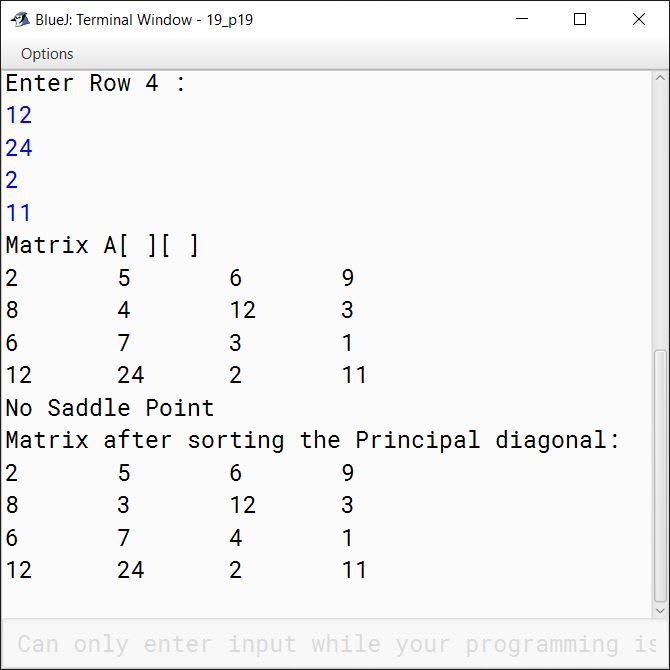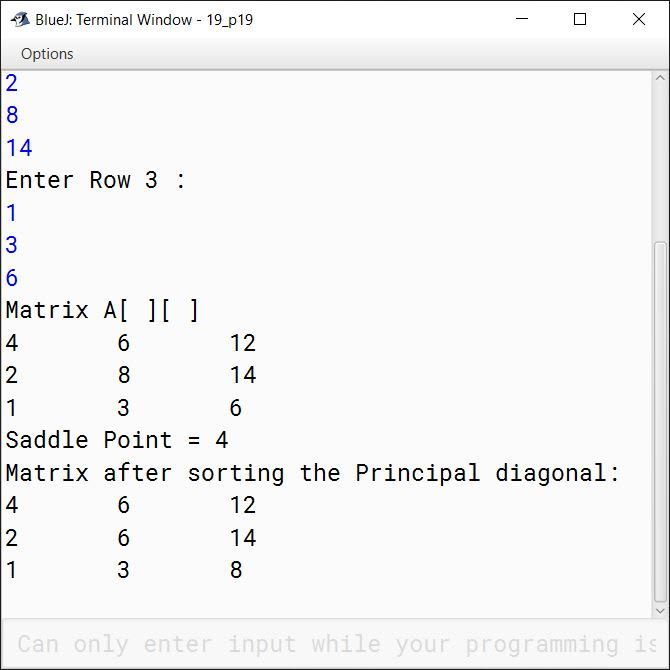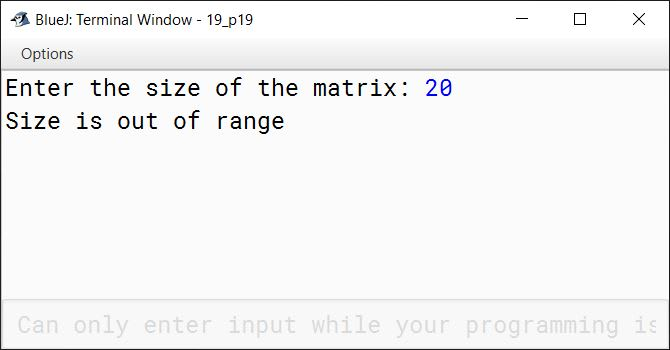#### Question 20

Write a program to input N and M number of names in two different single dimensional arrays A and B respectively, such that none of them have duplicate names. Merge the arrays A and B into a single array C, such that the resulting array is sorted alphabetically. Display all the three arrays.

Test your program for the following data and some random data:

Sample data:

Input:
Enter the names in array A, N = 2
Enter the names in array B, M = 3
First array: A
Suman
Anil
Second array: B
Usha
Sachin
John

Output:
Sorted Merged array: C
Anil
John
Sachin
Suman
Usha
Sorted First array: A
Anil
Suman
Sorted Second array: B
John
Sachin
Usha

Solution

```import java.util.Scanner;

public class KboatSDANames
{
public static void main(String args[]) {
Scanner in = new Scanner(System.in);
System.out.print("Enter the names in array A, N = ");
int n = in.nextInt();
System.out.print("Enter the names in array B, M = ");
int m = in.nextInt();
in.nextLine();

String a[] = new String[n];
String b[] = new String[m];
String c[] = new String[m+n];

System.out.println("First array: A");
for (int i = 0; i < n; i++) {
String name = in.nextLine();
for (int j  = i - 1; j >= 0; j--) {
if (a[j].equalsIgnoreCase(name)) {
System.out.println(name + " already present in array");
return;
}
}

a[i] = name;
}

System.out.println("Second array: B");
for (int i = 0; i < m; i++) {
String name = in.nextLine();

for (int k = 0; k < n; k++) {
if (a[k].equalsIgnoreCase(name)) {
System.out.println(name + " already present in array");
return;
}
}

for (int j = i - 1; j >= 0; j--) {
if (b[j].equalsIgnoreCase(name)) {
System.out.println(name + " already present in array");
return;
}
}

b[i] = name;
}

sortArray(a);
sortArray(b);
int aIdx = 0, bIdx = 0;

//Merge the arrays preserving the sorting
for (int i = 0; i < m+n; i++) {
if (aIdx == n || a[aIdx].compareToIgnoreCase(b[bIdx]) > 0) {
c[i] = b[bIdx];
bIdx++;
}
else if (bIdx == m || b[bIdx].compareToIgnoreCase(a[aIdx]) > 0) {
c[i] = a[aIdx];
aIdx++;
}
}

System.out.println("Sorted Merged array: C");
printArray(c);

System.out.println("Sorted First array: A");
printArray(a);

System.out.println("Sorted Second array: B");
printArray(b);
}

public static void sortArray(String arr[]) {
for (int i = 0; i < arr.length; i++) {
for (int j = 1; j < arr.length - i; j++) {
if (arr[j - 1].compareToIgnoreCase(arr[j]) > 0) {
String t = arr[j - 1];
arr[j - 1] = arr[j];
arr[j] = t;
}
}
}
}

public static void printArray(String arr[]) {
for (int i = 0; i < arr.length; i++) {
System.out.println(arr[i]);
}
}
}```
##### Output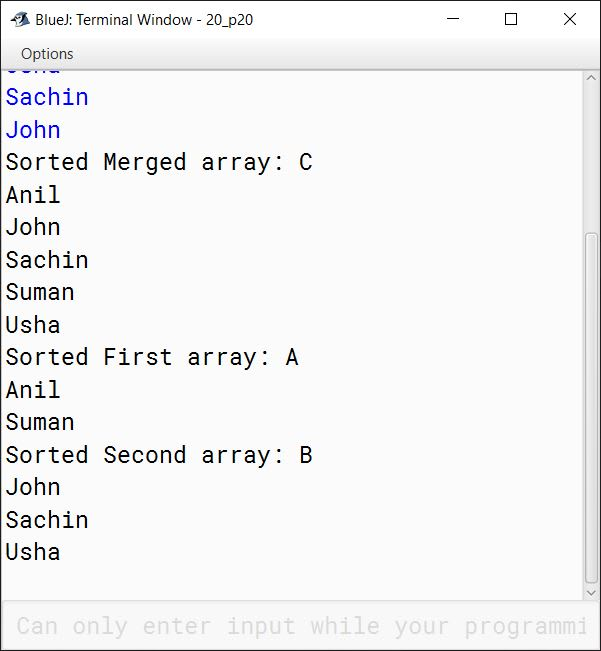#### Question 21

Numbers have different representations depending on the bases on which they are expressed. For example, in base 3, the number 12 is written as 110 (1 x 32 + 1 x 31 + 0 x 30) but base 8 it is written as 14 (1 x 81 + 4 x 80).

Consider for example, the integers 12 and 5. Certainly these are not equal if base 10 is used for each. But suppose, 12 was a base 3 number and 5 was a base 6 number then, 12 base 3 = 1 x 31 + 2 x 30, or 5 base 6 or base 10 (5 in any base is equal to 5 base 10). So, 12 and 5 can be equal if you select the right bases for each of them.

Write a program to input two integers x and y and calculate the smallest base for x and smallest base for y (likely different from x) so that x and y represent the same value. The base associated with x and y will be between 1 and 20 (both inclusive). In representing these numbers, the digits 0 to 9 have their usual decimal interpretations. The upper case letters from A to J represent digits 10 to 19 respectively.

Test your program for the following data and some random data.

Sample Data

Input:
x=12, y=5

Output:
12 (base 3)=5 (base 6)

Input:
x=10, y=A

Output:
10 (base 10)=A (base 11)

Input:
x=12, y=34

Output:
12 (base 8) = 34 (base 2)
12 (base 17) = 34 (base 5)
[∵ 34 (base 2) is not valid as only 0 & 1 are allowed in base 2]

Input:
x=123, y=456

Output:
123 is not equal to 456 in any base between 2 to 20

Input:
x=42, y=36

Output:
42 (base 7) = 36 (base 8)

Solution

```import java.util.Scanner;

public class KboatFindBase
{
public static void main(String args[]) {
Scanner in = new Scanner(System.in);
System.out.print("Enter x: ");
String x = in.nextLine();
System.out.print("Enter y: ");
String y = in.nextLine();

int xMax = getHighestDigit(x);
int yMax = getHighestDigit(y);

boolean found = false;
int decimalArr[] = new int;

for (int i = xMax; i < 20; i++) {
decimalArr[i] = convertToDecimal(x, i+1);
}

for (int i = yMax; i < 20; i++) {

int t = convertToDecimal(y, i+1);

for (int j = xMax; j < 20; j++) {
if (t == decimalArr[j]) {
found = true;
System.out.println(x + " (base " + (j+1) + ") = "
+ y + " (base " + (i+1) + ")");
break;
}
}

if (found) {
break;
}
}

if (!found) {
System.out.println(x + " is not equal to "
+ y + " in any base\nbetween 2 to 20");
}
}

public static int convertToDecimal(String numStr, int base) {
int num = 0;
int len = numStr.length();

for (int i = 0; i < len; i++) {
int mul = (int)Math.pow(base, len - i - 1);
char ch = numStr.charAt(i);
int d = digitValue(ch);
num += d * mul;
}

return num;
}

public static int digitValue(char c) {
int value = 0;

if (Character.isDigit(c)) {
value = c - '0';
}
else if (Character.isLetter(c) && c >= 'A' && c <= 'J') {
value = c - 'A' + 10;
}

return value;
}

public static int getHighestDigit(String numStr) {
int high = 0;
int len = numStr.length();

for (int i = 0; i < len; i++) {
char ch = numStr.charAt(i);
int d = digitValue(ch);
if (d > high) {
high = d;
}
}

return high;
}
}```
##### Output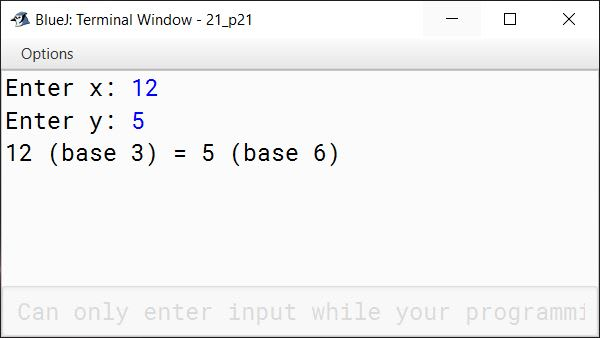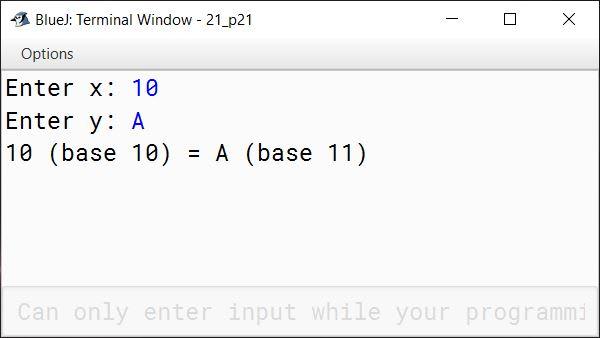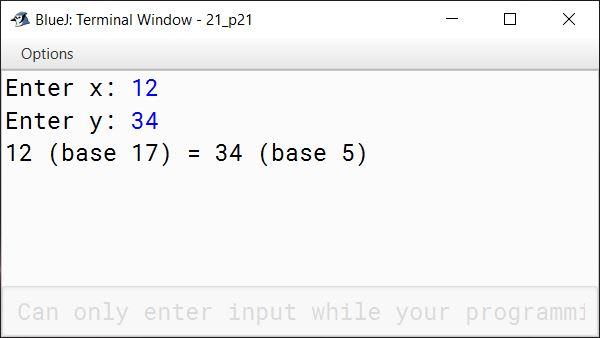#### Question 22

The manager of a company wants to analyze the machine usage from the records to find the utilization of the machine. He wants to know how long each user used the machine. When the user wants to use the machine, he must login to the machine and after finishing the work, he must logoff the machine.

Each log record consists of:

User Identification number
Logout time and date

Time consists of:

Hours
Minutes

Date consists of:

Day
Month

You may assume all logins and logouts are in the same year and there are 100 users at the most. The time format is 24 hours.

Design a program:

(a) To find the duration for which each user logged. Output all records along with the duration in hours (format hours: minutes).

(b) Output the record of the user who logged for the longest duration. You may assume that no user will login for more than 48 hours.

Test your program for the following data and some random data.

Sample Data

Input:
Number of users: 3
User Identification

Login Time and DateLogout Time and Date
20:1020-122:5021-12
12:3020-1212:3021-12
16:2020-1216:3020-12

Output:

User IdentificationLogin Time and DateLogout Time and DateDuration
Hours:Minutes
14920:1020-122:5021-126:40
17312:3020-1212:3021-1224:00
14216:2020-1216:3020-1200:10

The user who logged in for longest duration:

 173 12:30 20-12 12:30 21-12 24:00

Solution

```import java.util.Scanner;

public class KboatMachineUtilization
{
public static void main(String args[]) {
final int MINS_IN_DAY = 1440;
final int MINS_IN_HOUR = 60;
Scanner in = new Scanner(System.in);
System.out.print("Enter Number of Users: ");
int n = in.nextInt();
in.nextLine();

if (n > 100 || n < 1) {
System.out.println("Invalid Input!");
System.out.println("No of users must be between 1 and 100");
return;
}

String records[][] = new String[n];

int monthDays[] = {31, 28, 31, 30, 31, 30, 31, 31, 30, 31, 30, 31};

for (int i = 0; i < n; i++) {
System.out.println("Enter record of user " + (i+1) + ": ");
System.out.print("Enter User Identification: ");
records[i] = in.nextLine();
records[i] = in.nextLine();
records[i] = in.nextLine();
System.out.print("Enter Logout Time(hh:mm): ");
records[i] = in.nextLine();
System.out.print("Enter Logout Date(dd-mm): ");
records[i] = in.nextLine();
}
System.out.println();

int longIdx = 0;
int longDuration = 0;
for (int i = 0; i < n; i++) {
int duration = 0;
int tempIdx = records[i].indexOf(':');
int loginMin = Integer.parseInt(records[i].substring(tempIdx + 1));
tempIdx = records[i].indexOf(':');
int logoutHr = Integer.parseInt(records[i].substring(0, tempIdx));
int logoutMin = Integer.parseInt(records[i].substring(tempIdx + 1));
int m2 = logoutHr * MINS_IN_HOUR + logoutMin;

//If login & logout is on the same day
if (records[i].equals(records[i])) {
duration = m2 - m1;
}
else {
int daysDiff = 0;
tempIdx = records[i].indexOf('-');
int loginMonth = Integer.parseInt(records[i].substring(tempIdx + 1));
tempIdx = records[i].indexOf('-');
int logoutDay = Integer.parseInt(records[i].substring(0, tempIdx));
int logoutMonth = Integer.parseInt(records[i].substring(tempIdx + 1));
//If login & logout is in the same month
daysDiff = logoutDay - loginDay - 1;
}
else {
}

duration = (MINS_IN_DAY - m1) + m2 + daysDiff * MINS_IN_DAY;
}

if (duration > longDuration) {
longDuration = duration;
longIdx = i;
}

int durHr = duration / 60;
int durMin = duration % 60;
records[i] = (durHr == 0 ? "00" : durHr)
+ ":"
+ (durMin == 0 ? "00" : durMin);
}

System.out.println("Identification\tTime & Date\tTime & Date\tHours:Minutes");
for (int i = 0; i < n; i++) {
for (int j = 0; j < 6; j++) {
System.out.print(records[i][j] + "\t");
}
System.out.println();
}

System.out.println();
System.out.println("The user who logged in for longest duration:");
for (int j = 0; j < 6; j++) {
System.out.print(records[longIdx][j] + "\t");
}
}
}```
##### Output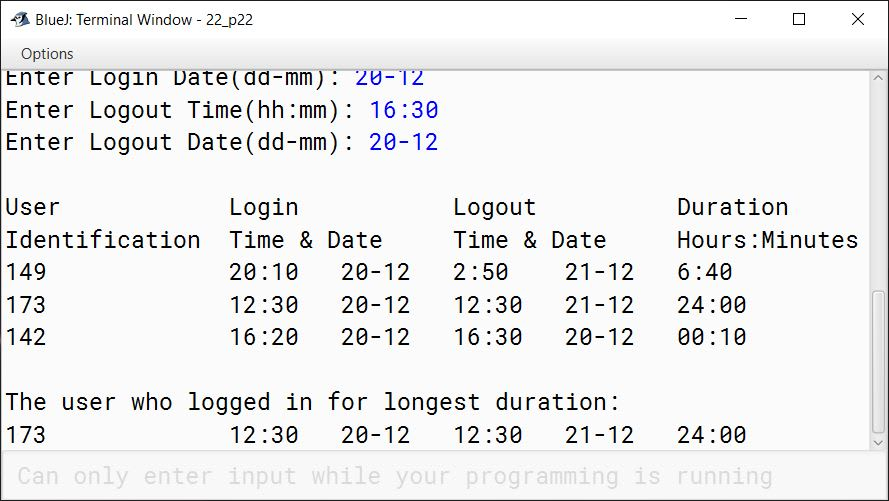#### Question 23

Write a program to accept a date in the string format dd/mm/yyyy and accept the name of the day on 1st of January of the corresponding year. Find the day for the given date.

Example:

Input:
Date: 5/7/2001
Day on 1st January : MONDAY

Output:
Day on 5/7/2001 : THURSDAY

Run the program on the following inputs:

Input DateDay on 1st JanuaryOutput day for
04/9/1998THURSDAYFRIDAY
31/8/1999FRIDAYTUESDAY
06/12/2000SATURDAYWEDNESDAY

The program should include the part for validating the inputs namely the date and day on 1st January of that year.

Solution

```import java.util.*;

public class KboatDayName
{

public static void main(String args[]) {
int monthDays[] = {31, 28, 31, 30, 31, 30, 31, 31, 30, 31, 30, 31};
String dayNames[] = {"MONDAY", "TUESDAY", "WEDNESDAY", "THURSDAY",
"FRIDAY", "SATURDAY", "SUNDAY"};
Scanner in = new Scanner(System.in);
System.out.print("Enter Date(dd/mm/yyyy): ");
String dateStr = in.nextLine();

StringTokenizer st = new StringTokenizer(dateStr, "/");
int tokenCount = st.countTokens();
if (tokenCount <= 0 || tokenCount > 3) {
System.out.println("Invalid Date");
return;
}

int day = Integer.parseInt(st.nextToken());
int month = Integer.parseInt(st.nextToken());
int year = Integer.parseInt(st.nextToken());

boolean leapYear = isLeapYear(year);
if (leapYear) {
monthDays = 29;
}

if (month < 1 || month > 12) {
System.out.println("Invalid Month");
return;
}

if (day < 1 || day > monthDays[month - 1]) {
System.out.println("Invalid Day");
return;
}

System.out.print("Day on 1st January: ");
String startDayName = in.nextLine();

int startDayIdx = -1;
for (int i = 0; i < dayNames.length; i++) {
if (dayNames[i].equalsIgnoreCase(startDayName)) {
startDayIdx = i;
break;
}
}

if (startDayIdx == -1) {
System.out.println("Invalid Day Name");
return;
}

//Calculate total days
int tDays = 0;
for (int i = 0; i < month - 1; i++) {
tDays += monthDays[i];
}

tDays += day;

int currDayIdx = tDays % 7 + startDayIdx - 1;
if (currDayIdx >= 7) {
currDayIdx -= 7;
}

System.out.println("Day on " + dateStr + " : " + dayNames[currDayIdx]);
}

public static boolean isLeapYear(int y) {
boolean ret = false;

if (y % 400 == 0) {
ret = true;
}
else if (y % 100 == 0) {
ret = false;
}
else if (y % 4 == 0) {
ret = true;
}
else {
ret = false;
}

return ret;
}
}```
##### Output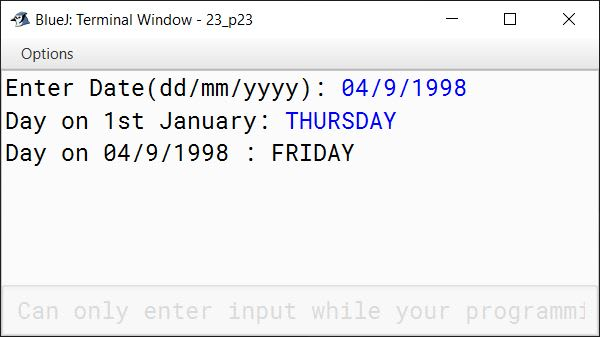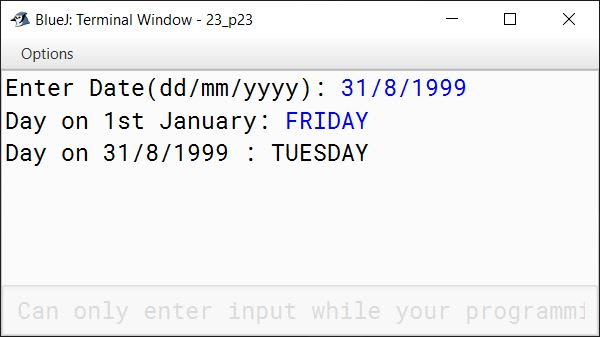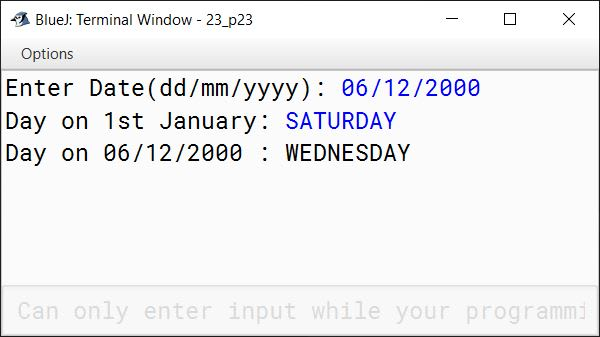#### Question 24

A wondrous square is an n by n grid which fulfils the following conditions:

1. It contains integers from 1 to n2, where each integer appears only once.
2. The sum of integers in any row or column must add up to 0.5 x n x (n2 + 1).

For example, the following grid is a wondrous square where the sum of each row or column is 65 when n=5.

 17 24 1 8 15 23 5 7 14 16 4 6 13 20 22 10 12 19 21 3 11 18 25 2 9

Write a program to read n (2 <= n <= 10) and the values stored in these n by n cells and output if the grid represents a wondrous square.

Also output all the prime numbers in the grid along with their row index and column index as shown in the output. A natural number is said to be prime if it has exactly two divisors. For example, 2, 3, 5, 7, 11 The first element of the given grid i.e. 17 is stored at row index 0 and column index 0 and the next element in the row i.e. 24 is stored at row index 0 and column index 1.

Test your program for the following data and some random data:

Input:
n = 4

 16 15 1 2 6 4 10 14 9 8 12 5 3 7 11 13

Output:
Yes, it represents a wondrous square

PrimeRow IndexColumn Index
203
330
523
731
1132
1333
1501

Input:
n = 3

 1 2 4 3 7 5 8 9 6

Output:
Not a wondrous square

PrimeRow IndexColumn Index
201
310
512
711

Input:
n = 2

 2 3 3 2

Output: Not a wondrous square

PrimeRow IndexColumn Index
200
211
301
310

Solution

```import java.util.Scanner;

public class KboatWondrousSquare
{
public static void main(String args[]) {
Scanner in = new Scanner(System.in);
System.out.print("Enter n: ");
int n = in.nextInt();

if (n < 2 || n > 10) {
System.out.println("Invalid value of n!");
return;
}

int a[][] = new int[n][n];

System.out.println("Enter elements of the matrix: ");
for (int i = 0; i < n; i++) {
System.out.println("Enter Row "+ (i+1) + " :");
for (int j = 0; j < n; j++) {
a[i][j] = in.nextInt();
}
}

System.out.println("The Matrix is:");
for (int i = 0; i < n; i++) {
for (int j = 0; j < n; j++) {
System.out.print(a[i][j] + "\t");
}
System.out.println();
}

//Check Wondrous
int nSq = n * n;
double validSum = 0.5 * n * (nSq + 1);
boolean wondrous = isWondrous(a);

if (wondrous) {
System.out.println("Yes, it represents a wondrous square");
}
else {
System.out.println("Not a wondrous square");
}

//Print Prime Numbers
printPrime(a);
}

public static boolean isWondrous(int arr[][]) {
int n = arr.length;
int nSq = n * n;
double validSum = 0.5 * n * (nSq + 1);

/*
* seenArr is used to check that
* numbers are not repeated
*/
boolean seenArr[] = new boolean[nSq];

for (int i = 0; i < n; i++) {

int rSum = 0, cSum = 0;

for (int j = 0; j < n; j++) {
if (arr[i][j] < 1 || arr[i][j] > nSq) {
return false;
}

//Number is not distinct
if (seenArr[arr[i][j] - 1]) {
return false;
}

seenArr[arr[i][j] - 1] = true;

rSum += arr[i][j];
cSum += arr[j][i];
}

if (rSum != validSum || cSum != validSum) {
return false;
}
}

return true;
}

public static void printPrime(int arr[][]) {

int n = arr.length;

System.out.println("Prime\tRow Index\tColumn Index");

for (int i = 0; i < n; i++) {
for (int j = 0; j < n; j++) {
if (isPrime(arr[i][j])) {
System.out.println(arr[i][j] + "\t" + i + "\t\t" + j);
}
}
}
}

public static boolean isPrime(int num) {
int c = 0;

for (int i = 1; i <= num; i++) {
if (num % i == 0) {
c++;
}
}

return c == 2;
}
}```
##### Output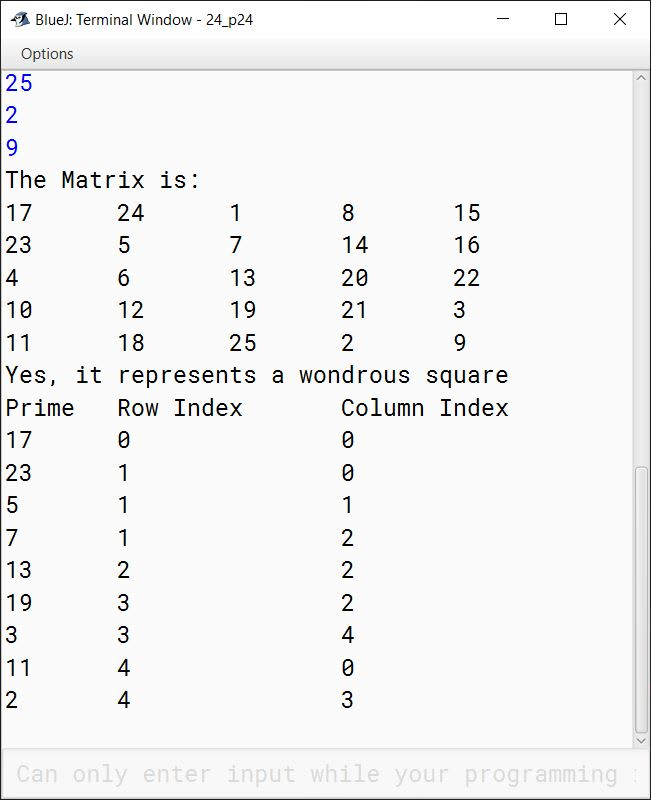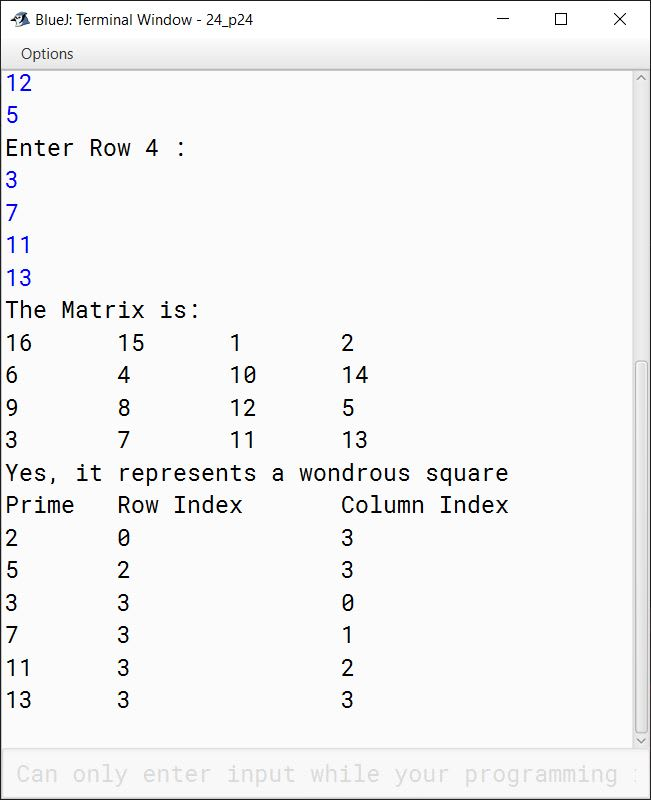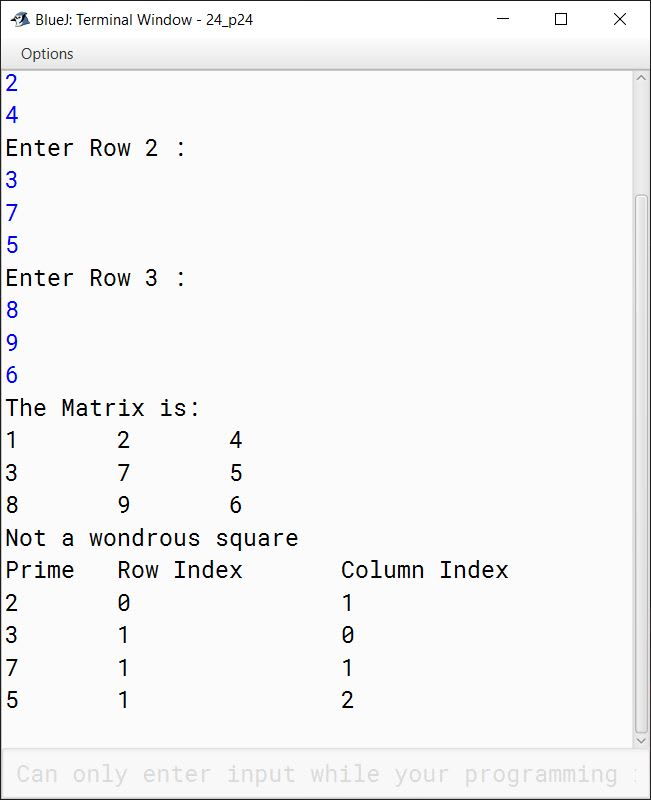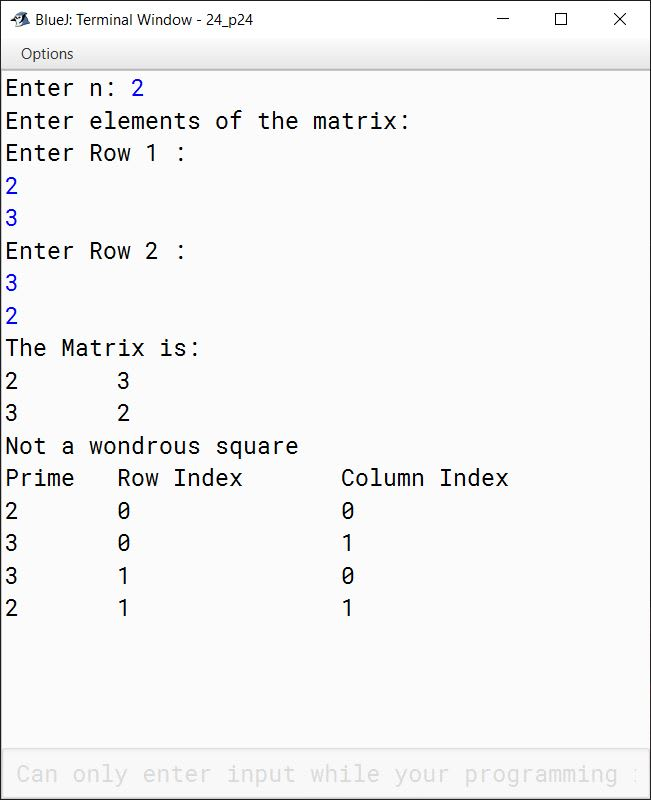#### Question 25

Write a program to input two valid dates, each comprising of Day (2 digits), Month (2 digits) and Year (4 digits) and calculate the days elapsed between both the dates.

Test your program for the following data values:

(a)
FIRST DATE:
Day: 24
Month: 09
Year: 1960

SECOND DATE:
Day: 08
Month: 12
Year: 1852

Output: xxxxxxxx
(these are actual number of days elapsed)

(b)
FIRST DATE:
Day: 10
Month: 01
Year: 1952

SECOND DATE:
Day: 16
Month: 10
Year: 1952

Output: xxxxxxxx
(these are actual number of days elapsed)

Solution

```import java.util.Scanner;

public class KboatDaysBetweenDates
{
public static void main(String args[]) {
Scanner in = new Scanner(System.in);
int d1[] = new int;
int d2[] = new int;
System.out.println("Enter First Date: ");
System.out.print("Enter Day: ");
d1 = in.nextInt();
System.out.print("Enter Month: ");
d1 = in.nextInt();
System.out.print("Enter Year: ");
d1 = in.nextInt();
System.out.println("Enter Second Date: ");
System.out.print("Enter Day: ");
d2 = in.nextInt();
System.out.print("Enter Month: ");
d2 = in.nextInt();
System.out.print("Enter Year: ");
d2 = in.nextInt();

int monthDays[] = {31, 28, 31, 30, 31, 30,
31, 31, 30, 31, 30, 31};

int n1 = d1 * 365 + d1;
int n2 = d2 * 365 + d2;

for (int i = 0; i < d1 - 1; i++) {
n1 += monthDays[i];
}

for (int i = 0; i < d2 - 1; i++) {
n2 += monthDays[i];
}

int y = d1 <= 2 ? d1 - 1 : d1;
n1 += y / 4 - y / 100 + y / 400;

y = d2 <= 2 ? d2 - 1 : d2;
n2 += y / 4 - y / 100 + y / 400;

int daysElapsed = Math.abs(n2 - n1);
System.out.println("No of days Elapsed = " + daysElapsed);
}
}```
##### Output